Comprehensive software for monitoring and evaluation of the economic situation of the enterprise and early warning# Wilcox ratio – net liquidation value

Method of calculation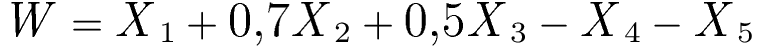where: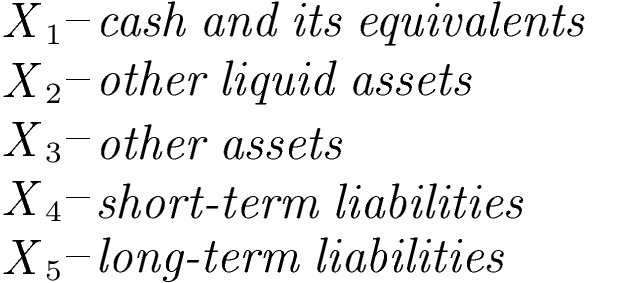Wilcox ratio description

The model was developed by W.Wilcox in 1970s and is widely recognized as the "Gambler's Ruin Approach".
The idea behind the model is to compute the net liquidation value of the firm. Based on the studies of financial performance of the firms that went bankrupt, Wilcox has established the coefficients of the liquidation value of assets:

• 100% for cash and cash equivalents,
• 70% for other liquid assets,
• 50% for other long-term assets.

Interpretation of the Wilcox ratio

• W < 0 -negative net liquid value of a firm means that the liabilities (debts) cannot be covered by the liquidated assets
• W > 0 -positive net liquid value of a firm means that the liabilities (debts) are covered by the liquidated assets
• The Wilcox ratio has interpretative power in the context of firm's ability to repay the current debts, as well as for the ability to cover the potential future liabilities. If the current Wilcox ratio is negative or close to 0 (as a threshold value), then the firm seems not able to launch new obligations. In a similar vein, a firm could easily compute how much of the additional debt it is able to cover by the currently hold assets. The positive value of Wilcox ratio indicates the maximum level of new credit (debt capacity).
• The main drawback of the Wilcox ratio is that it relies on the arbitrary coefficients determining the liquidation value of assets. In practice, there is a risk that the true liquidation value of assets could be lower than assumed in the Wilcox model.

Detailed decription of respective ratios and their impact on the Wilcox ratio value

X1 – cash and its equivalents

Method of calculation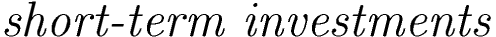Ratio's weight: +1.0
Units: times

X2 – other liquid assets

Method of calculation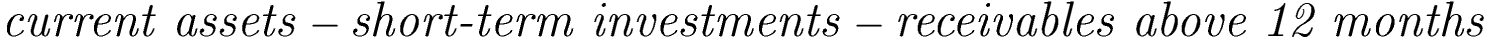Ratio's weight: +0.7
Units: times

X3 – other assets

Method of calculation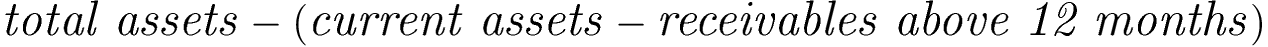Ratio's weight: +0.5
Units: times

X4 – short-term liabilities

Method of calculation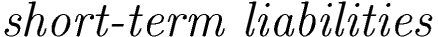Ratio's weight: -1.0
Units: times

X5 – long-term liabilities

Method of calculation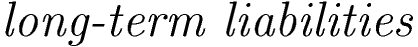Ratio's weight: -1.0
Units: times

We use cookies to ensure you have the best experience on our website. If you continue to use this site, we will assume that you are happy with it.